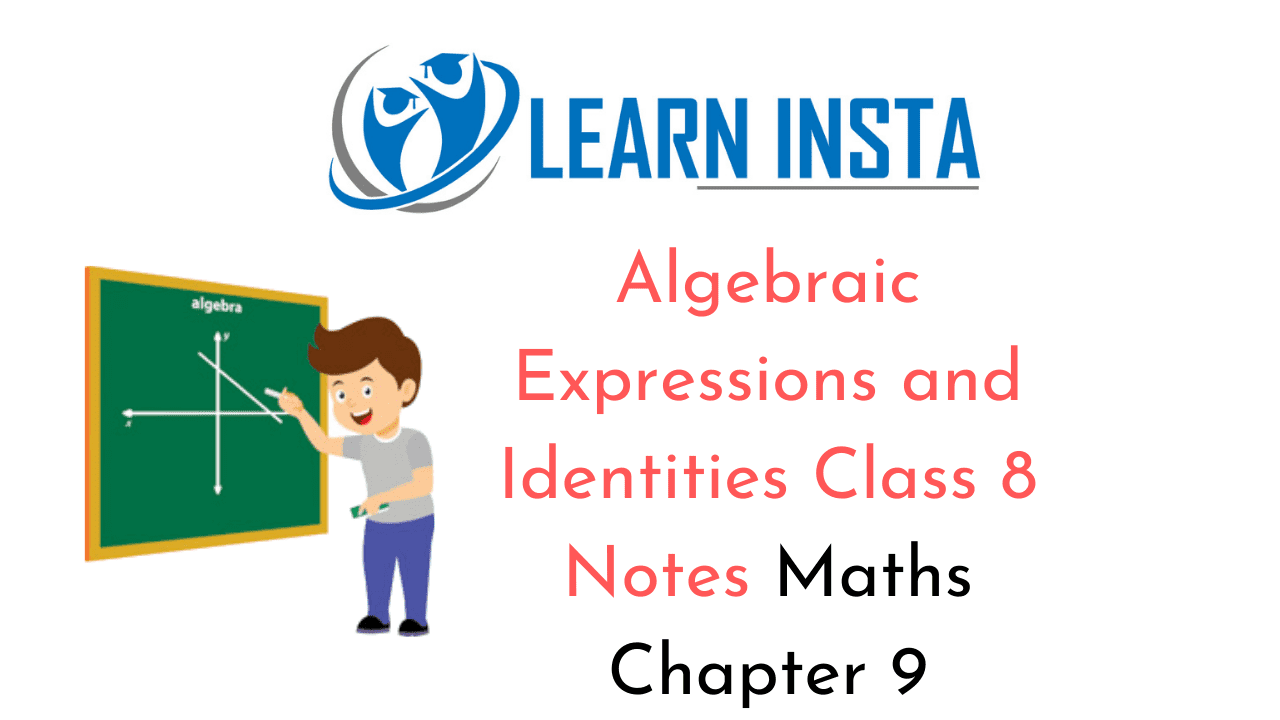On this page, you will find Algebraic Expressions and Identities Class 8 Notes Maths Chapter 9 Pdf free download. CBSE NCERT Class 8 Maths Notes Chapter 9 Algebraic Expressions and Identities will seemingly help them to revise the important concepts in less time.

## CBSE Class 8 Maths Chapter 9 Notes Algebraic Expressions and Identities

### Algebraic Expressions and Identities Class 8 Notes Conceptual Facts

1. Algebraic Expression: A combination of numbers which includes literal number connected by the
symbols +, -, x and + is called an algebraic expression.

For example: 5x, 8x -3, 2x + 3y, $$\frac{3}{4}$$x2 4xyz are some algebraic expressions.
Here. 5, 8, 3, 2. and 4 are constants and the literal numbers are x, y and z.

The different parts of the expression are called terms.
5x, 8x, 2x, 3y, $$\frac{3 x^{2}}{4}$$ etc., are all the terms.

2. Coefficient: A coefficient is a multiplicative factor in some term of a polynomial. It is usually a number,
but may be only expression along.

For example in 7x2 – 3xy + y + 3. The first three terms respectively have coefficient 7, -3 and 3 is a
constant in given polynomial.

3. Monomial: The expression having only one term is called monomial.
For example: 3x, 8xy, 6×2, 11xyz, etc.

4. Binomial: The expression containing two terms is called binomial.
Forexample: 2x +y,x +y, 3xy-5z, $$\frac{1}{2}$$ xy + 5, etc.

5. Trinomial: The expression containing three terms is called trinomial.
For example: x + 2y + 3, xy – z +$$\frac{1}{2}$$ , $$\frac{1}{2}$$ x2+ 2x + 5, etc.

6. Polynomial: Algebraic expression containing one or more terms with non-zero coefficient is called a
polynomial.
For example: 2+3x, x+y+3z-5, $$\frac{1}{2}$$ x2+yz -5, etc.

7. Like and Unlike Terms: Algebraic expressions having same combination of literal numbers are called
like terms.
For example: 4xy, -5xy, –$$\frac{17}{3}$$ xy, are like terms.

8. Algebraic expressions having different combinations of literal numbers are called unlike terms.
For example: (xy, yz, zx), (2x2, – 5xy2, 7xyz), (3, – 5x, 7yz) etc.

9. Degree of Algebraic Expression: Highest power of the variable of an algebraic expression is called its degree.
For example: Degree of 3x2 – 7x + 5 is 2.

Addition or Subtraction of two or more polynomials:

• Collect the like terms together.
• Find the sum or difference of the numerical coefficients of these terms.

For example:
(i) Add: 2x2y3, -5x2y3 + $$\frac{11}{2}$$x2yz(ii) Subtract: (3x – 5) from (8x – 25)